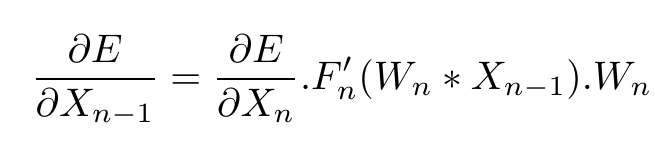This question might seem to be a bit theoretical and not suited as it is not directly linked to pytorch, but please bear with me as I have been asking this about and haven’t got a reply from anywhereWe know that during backpropagation, the weight update of the last layer is calculated by calculating the derivative of the loss with respect to the last layer’s weights and plugging in the differential values of the activation function and the inputs to the last layers. Now, when the (last-1) layer’s derivatives are calculated with the help of the derivatives of the inputs to the last layer ( and the output of the (last-1) layer), then are previous weight values of the last layer used to fill in the equation or the updated weight values used? In other words, are the weights of the last layer updated as soon as the gradients are calculated, or does it wait till calculating all the gradients of all the layers and then updates them simultaneously when we call optimizer.step() ?

For a more detailed view of the problem , please see–

Hi,

In pytorch, the weights are only updated when you do `optimizer.step()` which happens after the `.backward()` pass is completed. So no.
In general, if you rewrite all your formulas to add a `t` parameter to your weights to differentiate the version before the update and the one after. You will see that the gradient only ever depends on the original value, and never on the value after update (t+1). So if you compute the gradients, it should never happen in general, even if you fold the optimizer step into the backward pass.

1 Like

Thanks a lot! That cleared things upAnother small query if you will… in my Equation(2), I have the term as this:However, In “Efficient Backprop” by Yann Le Cun, (http://yann.lecun.com/exdb/publis/pdf/lecun-98b.pdf - Page 4 Equation 9), he has a transpose on the Wn term…and I can’t seem to understand why a transpose of the weights is being done…

This is most likely due to the differentiation rules depending on the convention you use.
You can check this by making sure the different matrix dimensions match.

1 Like

Oh… Okay thanksHi,
There is something else in this context which has been bothering me–
Suppose we have an input layer X0, weight W1, and output X1, then

1. X1.gradient is a scalar formed by summing up the gradients of all units of X1

2. W1 is a weight matrix containing internal weights w(i,j) which are updated based on :
* The scalar gradient of X1
* And the vector X0

This however, brings me up to the conclusion that for each w(i,j) to be updated individually, we should have the scalar X1 and each unit of the X0 matrix available for the specific i and j – Which is not plausible as the X0 matrix has a different shape as compared to the W1 matrix. How exactly are individual weights of the W1 matrix computed?

Hi,

Where does “X1.gradient is a *scalar formed by summing up the gradients of all units of X1” comes from?
The gradient for W is the outer product between the gradient of X1 (which is the same size as X1, not a scalar) and X0 which is is input.

1 Like

X1 is the output vector–and here’s what i found from that efficient backprop paper:

Here The scalar error at the node is not the gradient of the error with respect to X1?

Page 8 — 9th line of this

I think here, the “node” is a single element of X1. And so its gradient is just a single number.

Ah, but then if ẟ is not a single number, that brings down the whole concept of ‘Zigzagging’, right?–As the weight vectors are actually not changing together in ‘fixed directions’. Their directions are unique as ẟ is a vector. But then, can you give me a pointer to an article or a paper which explains why normalizing is necessary without using this ‘Zigzagging’ reference as most of what i have come across uses this. ThanksI don’t think a proof exist for neural nets in general.
But this considers a simpler case with a single weight. And I guess they claim that it extends similarly when you have more layers/weights?

1 Like